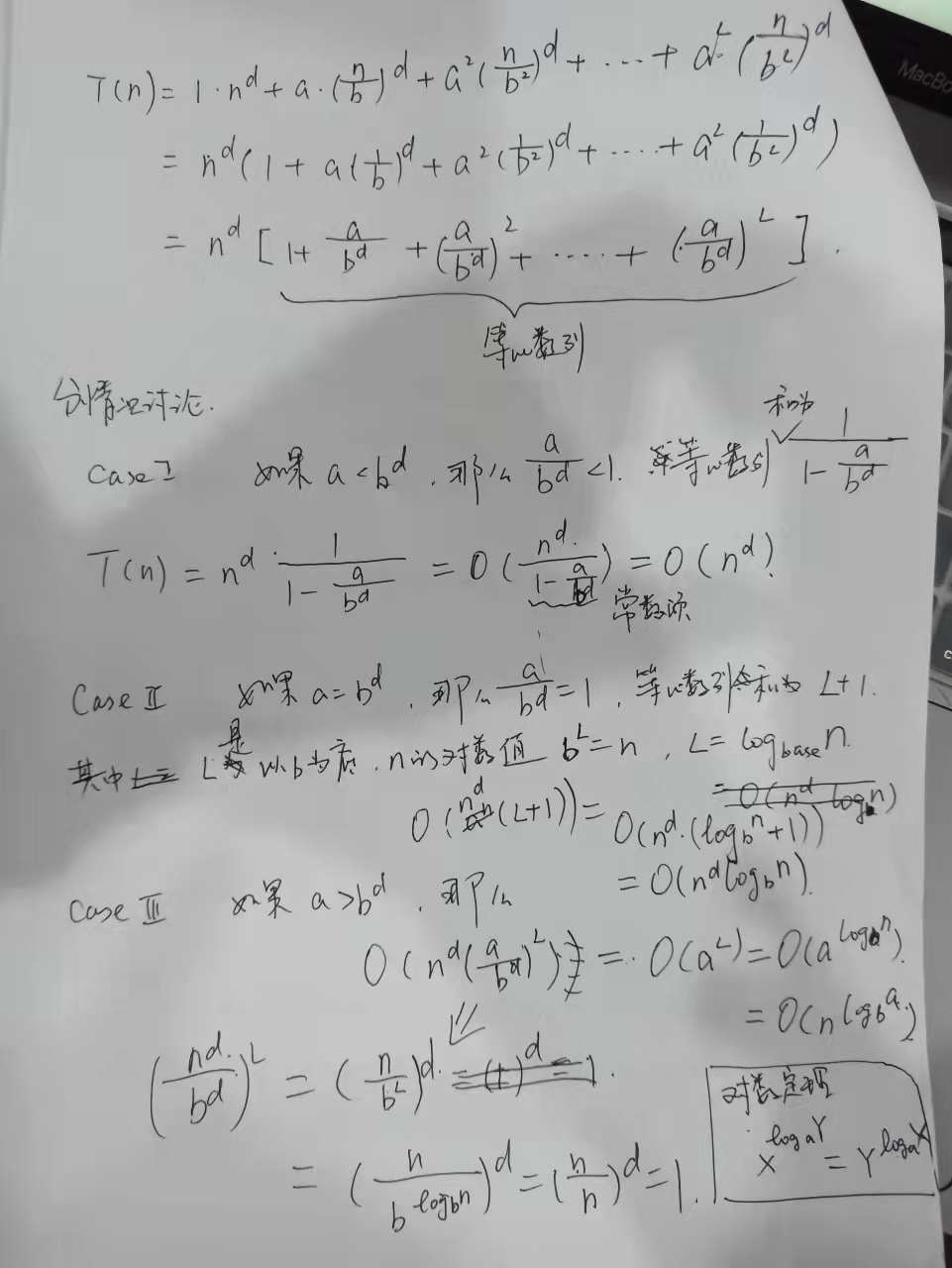## 抽象&分析

12=1+1+1……+1，一共12个1相加，积为1
12=2+2+2……+2，一共6个2相加，积为64
12=3+3+3+3，一共4个3相加，积为81
12=4+4+4，一共3个4相加，积为64
12=5+5+2，积为552，积为50
……

1. 拆一个正整数为n个正整数相乘，尽可能地多拆3出来，不能拆3，就拆2或者4，坚决不能拆1；
2. 如果拆出来的数比3大的越多，乘积就越小，比如上面的4^3和5%2等比3大的拆分方案；
知道了这个规律后，就非常容易判断1000^999和999^1000哪个数更大，显然，999比1000更接近3，999^1000更大。那么上面的规律如何证明呢，我记得老师噼里啪啦写了推导，证明过程如下：

N = x + x + …… + x，把大N拆成n个x的和，n=N/x，要使得f(x) = x^n=x^(N/x)最大。

## 联想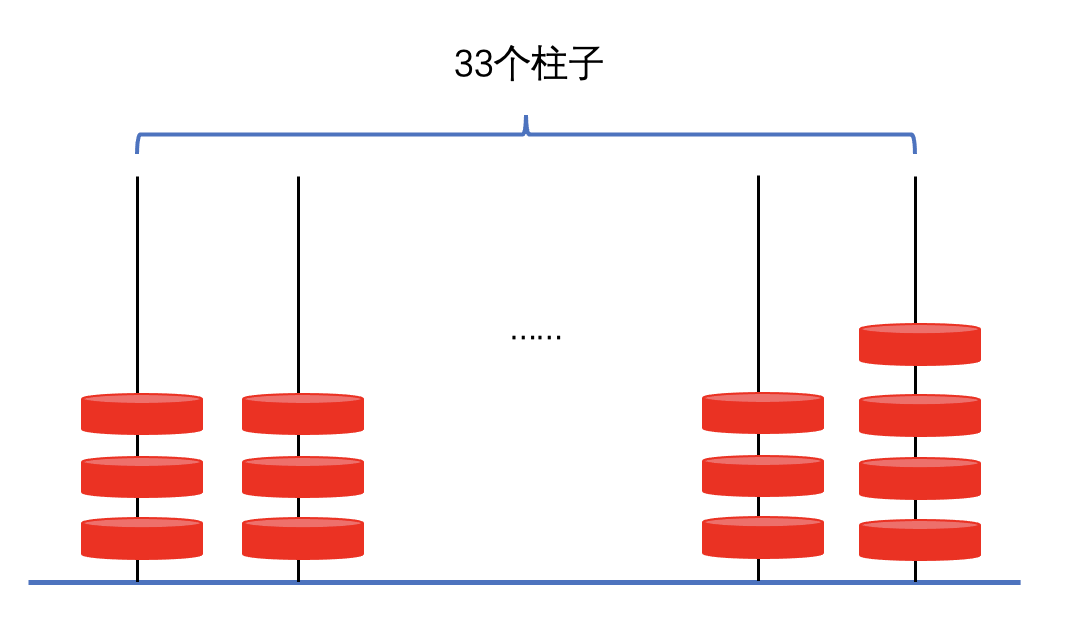## 问题

1. 0-1背包问题的三种实现，时间复杂度和空间复杂度分析；
2. 针对上述的代码实现，构建实际测试用例，看实际case下的benchmark数据；

## 讨论

0-1背包问题（NP-Complete）

``````func knapsack01C(v []int, w []int, W int) int {
defer time_cost(time.Now(), "knapsack01C")
r := 0
var help func(s int, c_w int, c_v int)
help = func(s int, c_w int, c_v int) {
if c_v > r {
r = c_v
}

for i := s; i < len(v); i++ {
if c_w+w[i] <= W {
help(i+1, c_w+w[i], c_v+v[i])
}
}
}

help(0, 0, 0)

return r
}
``````

v = {1, 2, 4, 5}
w = {1, 1, 2, 2}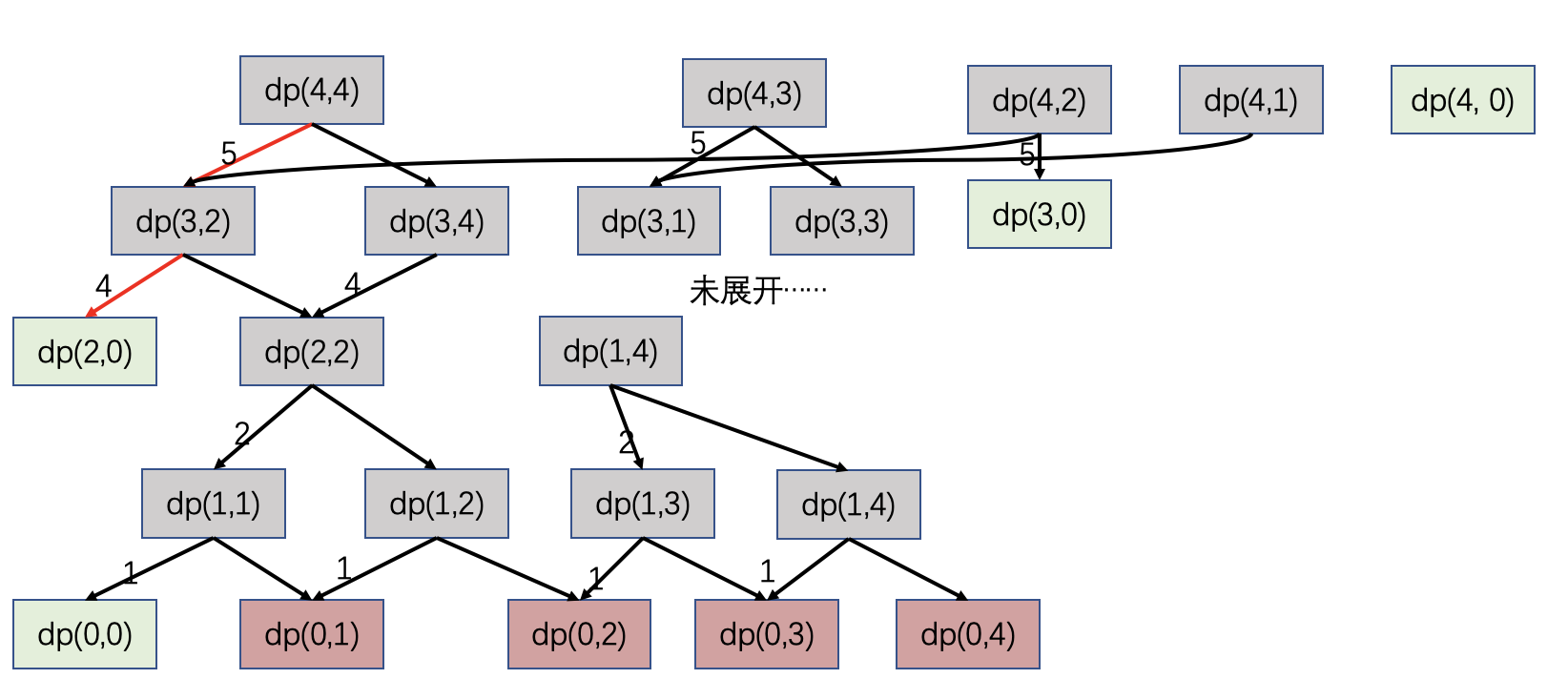``````func knapsack01R(v []int, w []int, W int) int {
defer time_cost(time.Now(), "knapsack01R")
n := len(v)
dp := make(map[int]int)
var help func(i int, l_w int) int
help = func(i int, l_w int) int {
if i == 0 {
return 0
}

k := int{i, l_w}
t, ok := dp[k]
if ok {
return t
}

l, r := 0, 0
if l_w >= w[i-1] {
l = help(i-1, l_w-w[i-1]) + v[i-1]
}
r = help(i-1, l_w)

ret := 0
if l > r {
ret = l
} else {
ret = r
}

dp[k] = ret
return ret
}

r := 0
for i := 0; i <= n; i++ {
t := help(i, W)
if t > r {
r = t
}
}
return r
}
``````

dpi = dpi-1 j < w[i] or max{dpi-1]+v[i], w[i] <= j <= W}
ans = max{dpN}

``````func knapsack01D(v []int, w []int, W int) int {
defer time_cost(time.Now(), "knapsack01D")
n := len(v)
var dp [][]int
for i := 0; i <= n; i++ {
row := make([]int, W+1)
dp = append(dp, row)
}

for i := 0; i <= n; i++ {
dp[i] = 0
}

for j := 0; j <= W; j++ {
dp[j] = 0
}

for i := 1; i <= n; i++ {
for j := 0; j <= W; j++ {
dp[i][j] = dp[i-1][j]
if j >= w[i-1] && dp[i-1][j-w[i-1]]+v[i-1] > dp[i][j] {
dp[i][j] = dp[i-1][j-w[i-1]] + v[i-1]
}
}
}

r := 0
for i := 0; i <= W; i++ {
if dp[n][i] > r {
r = dp[n][i]
}
}

return r
}``````

``````func knapsack01D2(v []int, w []int, W int) int {
defer time_cost(time.Now(), "knapsack01D")
n := len(v)
dp := make([]int, W+1)

for j := 0; j <= W; j++ {
dp[j] = 0
}

for i := 1; i <= n; i++ {
for j := W; j >= w[i-1]; j-- {
if dp[j-w[i-1]]+v[i-1] > dp[j] {
dp[j] = dp[j-w[i-1]] + v[i-1]
}
}
}

r := 0
for j := 0; j <= W; j++ {
if dp[j] > r {
r = dp[j]
}
}

return r
}``````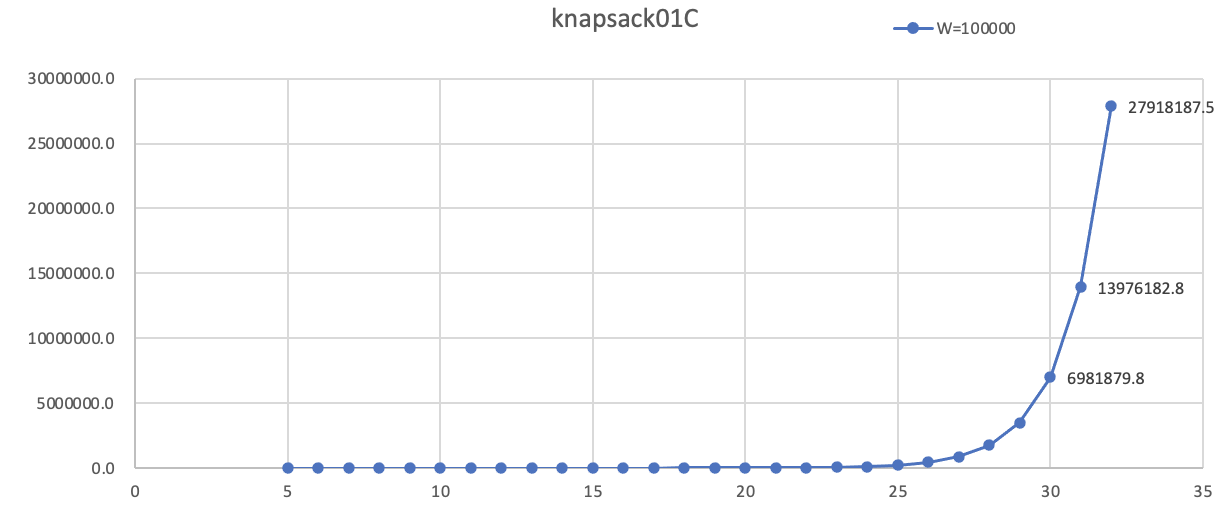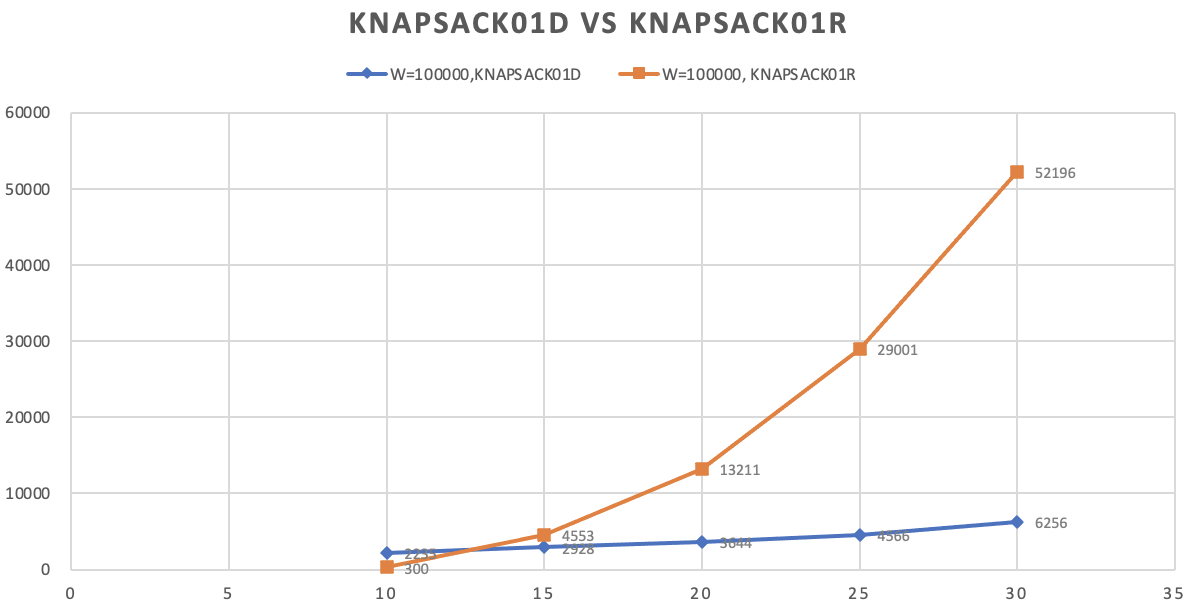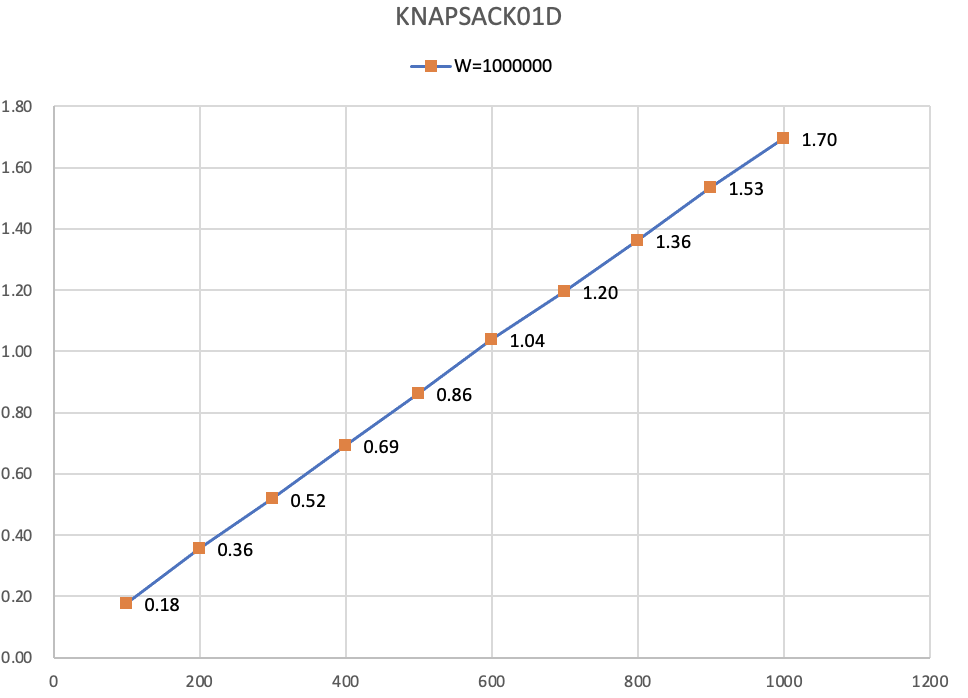## 证明过程

``````func reservoirSampling(sample int, total int) ([]int, int) {
r1 := make([]int, total)
r2 := 0

for i := 0; i < total; i++ {
r1[i] = i
}

for i := sample; i < total; i++  {
tmp := rand.Intn(i)
if tmp < sample {
r1[i], r1[tmp] = tmp, i
}
}

return r1[:sample], r2
}``````

1. 来看第1～k个数据，如果要保留到最后的采样集合中，一开始1~k保留的概率为1，直到k+1个数据出现，对于1~k个数据中任何一个数据来说，被选中换出的概率是1/(k+1)，反面就是被保留的概率，就是1-1/(k+1)=k/k+1，紧接着第k+2个数据来了，那么类似的分析法，被保留的概率是(k+1)/(k+2)，以此类推，知道第n个数据出现，概率就是(n-1)/n，前面分析的概率全部相乘，最终被保留的概率是k/n。
2. 来看第i个数据（i>k），第i个数据被选中的概率，显然是k/i，紧接着第i+1个数据来了，此时前面第i个数据被剔除的概率为1/(i+1)，被保留的概率就是1-1/(i+1)=i/(i+1)，第i+2个数据来了，类似的分析法，第i个数据被保留的概率是(i+1)/(i+2)，那么到第n个数据的时候，被保留的概率是(n-1)/n，前面分析的概率全部相乘，最终被保留的概率是k/n。
完美证明，如论是最开始的1~k个数据，还是k+1~n个数据，选中被保留到最后的概率都是k/n，是不是很神奇？简单的数学，总是令人开心，不需要关心一堆复杂公式的推导，哈哈。

## 和naive随机采样的对比

``````func naiveSampling(sample int, total int) ([]int, int) {
r1 := make([]int, sample)
r2 := 0
m := make(map[int]bool)
for i := 0; i < sample;  {
tmp := rand.Intn(total)
if _, ok := m[tmp]; ok {
// 不去重
r2++
// 去重
// continue
}

r1[i] = tmp
m[tmp] = true
i++
}

return r1, r2
}

func randomSequence(sample int, count int, total int, f func(int,int)([]int, int)) {
start := time.Now()
rand.Seed(time.Now().UnixNano())

m := make(map[int]int)
r := 0
for i := 0; i < count; i++ {
r1, r2 := f(sample, total)
r += r2
for _, v := range r1 {
m[v] += 1
}
}
elapsed := time.Since(start)
fmt.Printf("elapsed=%v\nr|t=%d|%d, rr=%f, sample=%d\nm=%v\n",
elapsed, r, sample*count, float32(r)/float32(sample*count), len(m), m)
}

func main() {
fmt.Printf("naiveSampling, sample=500, total=3000\n")
randomSequence(500, 10000, 3000, naiveSampling)
fmt.Printf("reservoirSampling, sample=500, total=3000\n")
randomSequence(500, 10000, 3000, reservoirSampling)

fmt.Printf("naiveSampling, sample=1000, total=3000\n")
randomSequence(1000, 10000, 3000, naiveSampling)
fmt.Printf("reservoirSampling, sample=1000, total=3000\n")
randomSequence(1000, 10000, 3000, reservoirSampling)
}``````

``````naiveSampling, sample=500, total=3000
elapsed=759.6145ms
r|t=394722|5000000, rr=0.078944, sample=3000
m=map[0:1730 1:1697 2:1624 3:1658 4:1664 5:1673 6:1650 7:1672 8:1679 9:1678 10:1668 11:1652 12:1630 13:1661 14:1682 ……]
reservoirSampling, sample=500, total=3000
elapsed=835.8041ms
r|t=0|5000000, rr=0.000000, sample=3000
m=map[0:1585 1:1569 2:1618 3:1682 4:1631 5:1549 6:1646 7:1721 8:1709 9:1610 10:1699 11:1657 12:1645 13:1692 14:1684 ……]
naiveSampling, sample=1000, total=3000
elapsed=1.4001243s
r|t=1494500|10000000, rr=0.149450, sample=3000
m=map[0:3286 1:3222 2:3372 3:3338 4:3302 5:3365 6:3303 7:3403 8:3419 9:3299 10:3315 11:3254 12:3454 13:3362 14:3317 ……]
reservoirSampling, sample=1000, total=3000
elapsed=894.9584ms
r|t=0|10000000, rr=0.000000, sample=3000
m=map[0:3249 1:3393 2:3280 3:3322 4:3276 5:3417 6:3217 7:3345 8:3305 9:3444 10:3299 11:3365 12:3411 13:3276 14:3339 ……]``````

## 总结

1. 假设有K台机器，将大数据集分成K个数据流，每台机器使用单机版蓄水池抽样处理一个数据流，抽样m个数据，并最后记录处理的数据量为N1, N2, ..., NK(假设m<Nk)。N1+N2+...+NK=N。
2. 取[1, N]一个随机数d，若d<N1，则在第一台机器的蓄水池中等概率不放回地（1/m）选取一个数据；若N1<=d<(N1+N2)，则在第二台机器的蓄水池中等概率不放回地选取一个数据；以此类推，重复m次，则最终从N大数据集中选出m个数据。

## 另类的字符串比较

``````boolean safeEqual(String a, String b) {
if (a.length() != b.length()) {
return false;
}
int equal = 0;
for (int i = 0; i < a.length(); i++) {
equal |= a.charAt(i) ^ b.charAt(i);
}
return equal == 0;
}
``````

``````func equals(a, b string) bool {
if len(a) != len(b) {
return false
}

for i := 0; i < len(a); i++ {
if a[i] != b[i] {
return false
}
}

return true
}
``````

## 计时攻击（Timing Attack）

``````bool verify(message, digest) {
my_digest = HMAC(key, message);
return my_digest.equals(digest) ;
}
``````

``````0 0.005450913
1 0.005829198
2 0.004905407
3 0.005286876
4 0.005597611
5 0.004814430
6 0.004969118
7 0.005335884
8 0.004433182
9 0.004440246
a 0.004860263
b 0.004561121
c 0.004463188
d 0.004406799
e 0.004978907
f 0.004887240
``````

``````{
"0"=>[7, 1, 3, 3, 15, 5, 4, 9, 15, 10, 13, 2, 14, 9, 4, 14, 7, 9, 15, 2, 14, 9, 14, 6, 11...],
"1"=>[13, 4, 7, 11, 0, 4, 0, 2, 14, 11, 6, 7, 2, 2, 14, 11, 8, 10, 5, 13, 11, 7, 4, 9, 3...],
"2"=>[14, 5, 15, 5, 1, 0, 3, 1, 9, 12, 4, 4, 1, 1, 8, 6, 9, 4, 9, 5, 8, 3, 12, 8, 5...],
"3"=>[15, 2, 9, 7, 2, 1, 14, 11, 7, 8, 8, 1, 4, 7, 12, 15, 13, 0, 4, 1, 7, 0, 3, 0, 0...],
"4"=>[12, 10, 14, 15, 8, 9, 10, 12, 10, 4, 1, 13, 15, 15, 3, 1, 6, 8, 2, 6, 15, 4, 0, 3, 2...],
"5"=>[5, 13, 13, 12, 7, 8, 13, 14, 3, 13, 2, 12, 7, 14, 2, 10, 12, 5, 8, 0, 4, 10, 5, 10, 12...]
"6"=>[0, 15, 11, 13, 5, 15, 8, 8, 4, 7, 12, 9, 10, 11, 11, 7, 0, 6, 0, 9, 2, 6, 15, 13, 14...]
"7"=>[1, 9, 0, 10, 6, 6, 2, 4, 12, 9, 5, 10, 5, 10, 7, 2, 4, 14, 6, 7, 13, 11, 6, 12, 4...],
"8"=>[4, 0, 2, 1, 9, 11, 12, 13, 11, 14, 0, 15, 9, 0, 0, 13, 11, 13, 1, 8, 6, 5, 11, 15, 7...],
"9"=>[11, 11, 10, 4, 13, 7, 6, 3, 2, 2, 14, 5, 3, 3, 15, 9, 14, 7, 10, 3, 0, 14, 1, 5, 15...],
"a"=>[8, 3, 6, 14, 10, 2, 7, 5, 1, 3, 3, 0, 0, 6, 10, 12, 15, 12, 12, 15, 9, 13, 13, 11, 9...],
"b"=>[9, 12, 5, 8, 3, 3, 5, 15, 0, 6, 11, 11, 12, 8, 1, 3, 1, 11, 11, 14, 5, 1, 2, 1, 6...],
"c"=>[6, 7, 8, 2, 12, 10, 9, 10, 6, 1, 10, 8, 6, 4, 6, 4, 3, 2, 7, 11, 1, 8, 7, 2, 13...],
"d"=>[2, 14, 4, 0, 14, 12, 11, 0, 8, 0, 15, 3, 8, 12, 5, 0, 10, 1, 3, 4, 12, 12, 8, 14, 8...],
"e"=>[10, 8, 12, 6, 11, 13, 1, 6, 13, 5, 7, 14, 11, 5, 9, 5, 2, 15, 14, 10, 10, 2, 10, 4, 1...],
"f"=>[3, 6, 1, 9, 4, 14, 15, 7, 5, 15, 9, 6, 13, 13, 13, 8, 5, 3, 13, 12, 3, 15, 9, 7, 10...]
}
``````

``````"f", 5.302
"0", 7.17
"6", 7.396
"3", 7.472
"5", 7.562
"a", 7.602
"2", 7.608
"8", 7.626
"9", 7.688
"b", 7.698
"1", 7.704
"e", 7.812
"4", 7.82
"d", 7.826
"7", 7.854
"c", 7.86
``````

``````计时攻击往往用于攻击一些性能较弱的计算设备，例如一些智能卡。我们通过实验发现，也能用于攻击普通的软件系统。本文通过实验证明，通过这种计时攻击方式能够攻破一个基于 OpenSSL 的 web 服务器的私钥。结果证明计时攻击用于进行网络攻击在实践中可行的，因此各大安全系统需要抵御这种风险。
``````

## 理论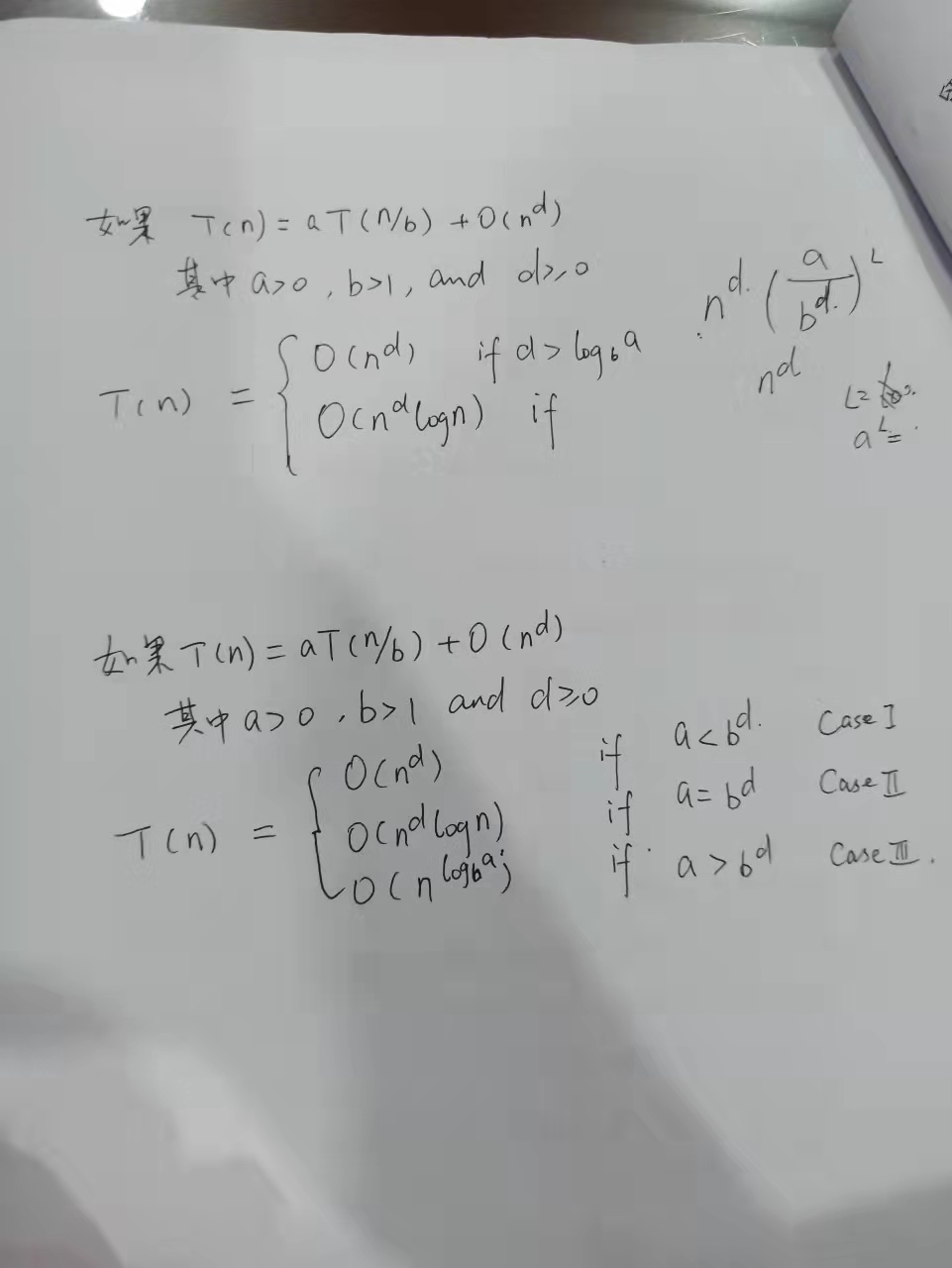## 证明